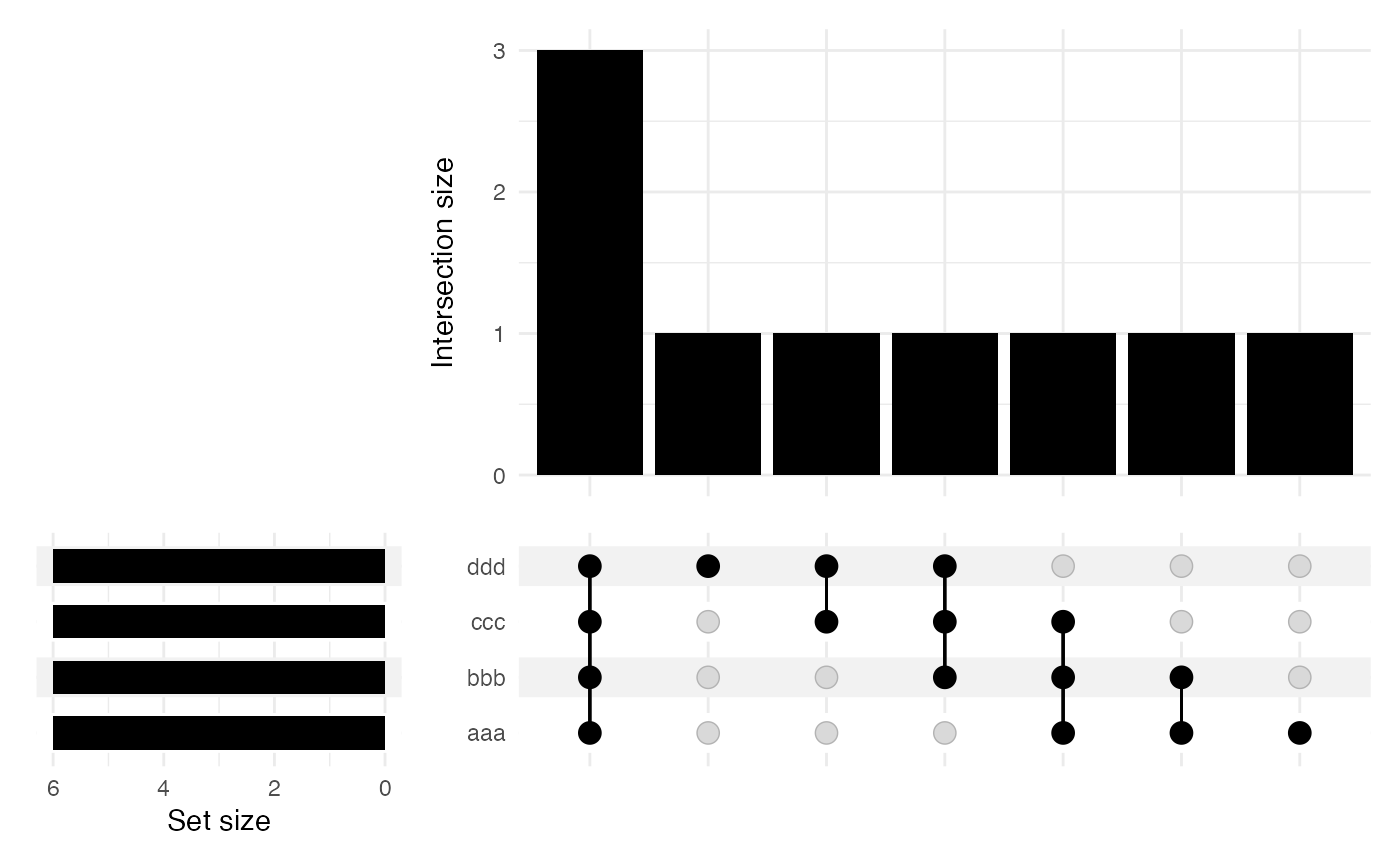UpSet plot

## Usage

plotUpset(object, ...)

# S4 method for DFrame
plotUpset(object, ...)

# S4 method for data.frame
plotUpset(object, ...)

# S4 method for list
plotUpset(object, ...)

# S4 method for matrix
plotUpset(
object,
minSize = 0L,
maxSize = Inf,
nIntersections = 40L,
orderBySize = c(intersections = TRUE, sets = TRUE),
labels = list(title = NULL, subtitle = NULL)
)

## Arguments

object

Object.

...

minSize, maxSize

Non-negative integer(1) or Inf. Minimal/maximal number of observations in an intersection for it to be included. Defaults to all observations. Note that maxSize must be greater than minSize.

nIntersections

integer(1) or Inf. Maximum number of intersections to plot. Set Inf to plot all intersections.

orderBySize

logical. Whether to order main bar plot and/or intersection matrix by set size.

• "intersection": refers to main intersection bar plot.

• "sets": refers to set intersection matrix, shown as connected dots. When TRUE, orders by the set size (see plot to the left).

Can pass in TRUE/FALSE boolean flag and both "intersection" and "sets" settings will inherit.

labels

list. ggplot2 labels. See ggplot2::labs() for details.

Plot.

## Details

S4 wrapper for ComplexUpset::upset() with improved default aesthetics.

## Note

Updated 2022-04-05.

• intersectionMatrix().

• ComplexUpset::upset().

• ComplexUpset::upset_themes.

• UpSetR package (legacy approach).

## Examples

## list ====
list <- list(
"aaa" = c("a", "b", "c", "d", "e", "f"),
"bbb" = c("b", "c", "d", "e", "f", "g"),
"ccc" = c("c", "d", "e", "f", "g", "h"),
"ddd" = c("d", "e", "f", "g", "h", "i")
)
print(list)
#> $aaa #>  "a" "b" "c" "d" "e" "f" #> #>$bbb
#>  "b" "c" "d" "e" "f" "g"
#>
#> $ccc #>  "c" "d" "e" "f" "g" "h" #> #>$ddd
#>  "d" "e" "f" "g" "h" "i"
#>
plotUpset(list)## matrix ====
mat <- AcidBase::intersectionMatrix(list)
print(mat)
#>     aaa   bbb   ccc   ddd
#> a  TRUE FALSE FALSE FALSE
#> b  TRUE  TRUE FALSE FALSE
#> c  TRUE  TRUE  TRUE FALSE
#> d  TRUE  TRUE  TRUE  TRUE
#> e  TRUE  TRUE  TRUE  TRUE
#> f  TRUE  TRUE  TRUE  TRUE
#> g FALSE  TRUE  TRUE  TRUE
#> h FALSE FALSE  TRUE  TRUE
#> i FALSE FALSE FALSE  TRUE
plotUpset(mat)